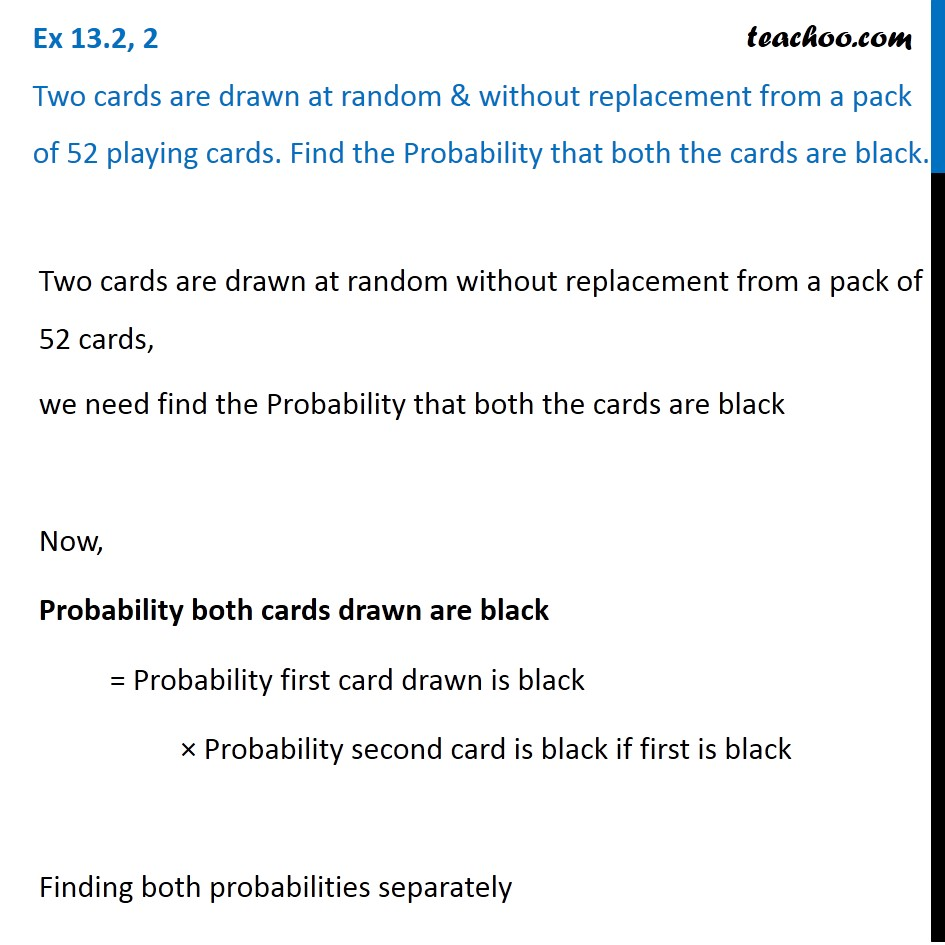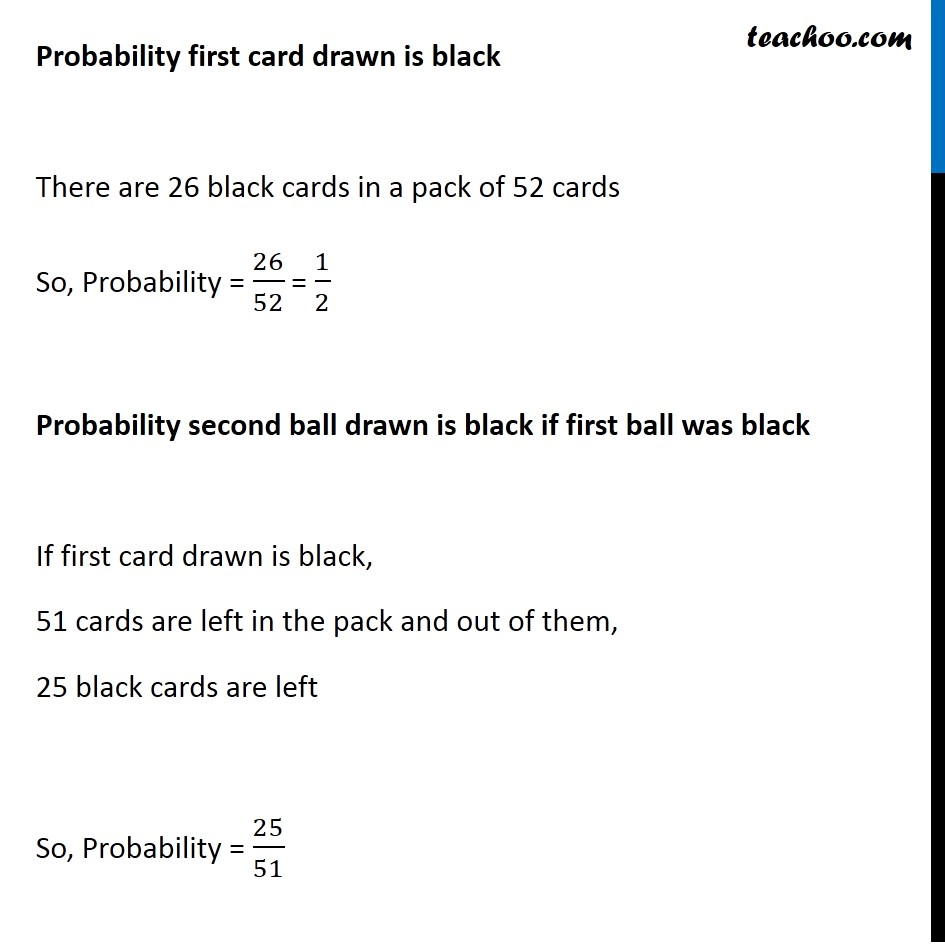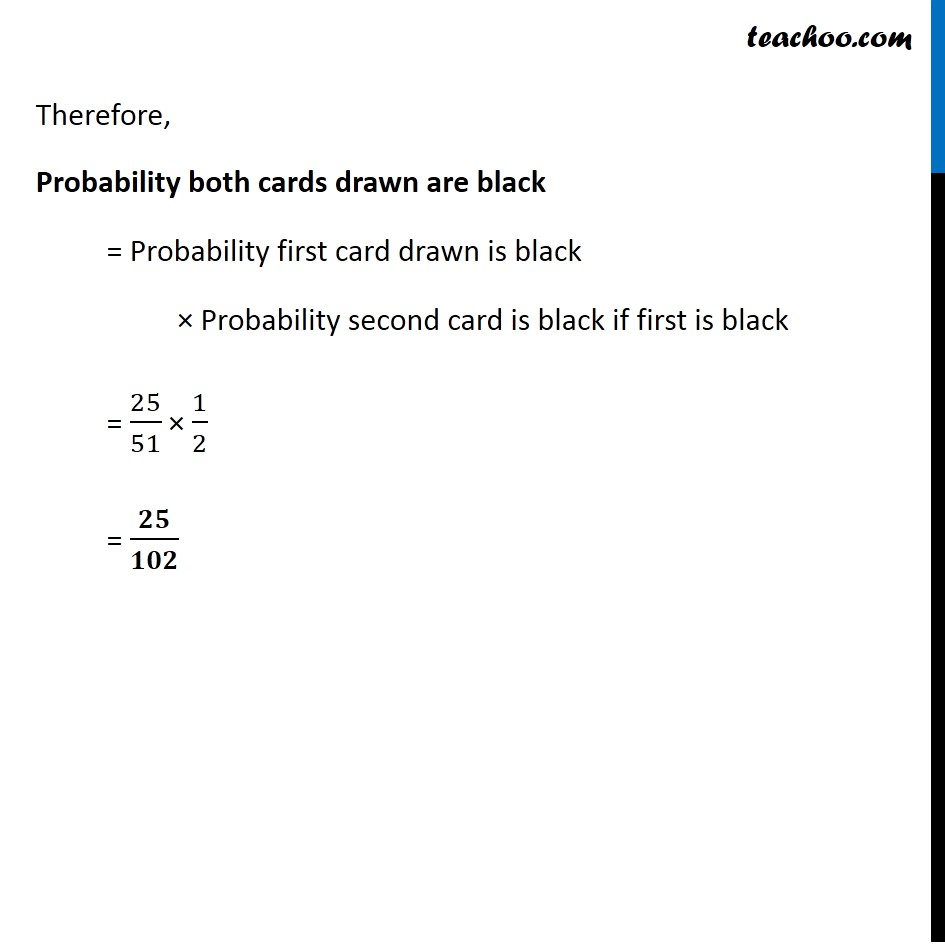Ex 13.2

Chapter 13 Class 12 Probability
Serial order wiseSolve all your doubts with Teachoo Black (new monthly pack available now!)

### Transcript

Ex 13.2, 2 Two cards are drawn at random & without replacement from a pack of 52 playing cards. Find the Probability that both the cards are black.Two cards are drawn at random without replacement from a pack of 52 cards, we need find the Probability that both the cards are black Now, Probability both cards drawn are black = Probability first card drawn is black × Probability second card is black if first is black Finding both probabilities separately Probability first card drawn is black There are 26 black cards in a pack of 52 cards So, Probability = 26/52 = 1/2 Probability second ball drawn is black if first ball was black If first card drawn is black, 51 cards are left in the pack and out of them, 25 black cards are left So, Probability = 25/51 Therefore, Probability both cards drawn are black = Probability first card drawn is black × Probability second card is black if first is black = 25/51 × 1/2 = 𝟐𝟓/𝟏𝟎𝟐